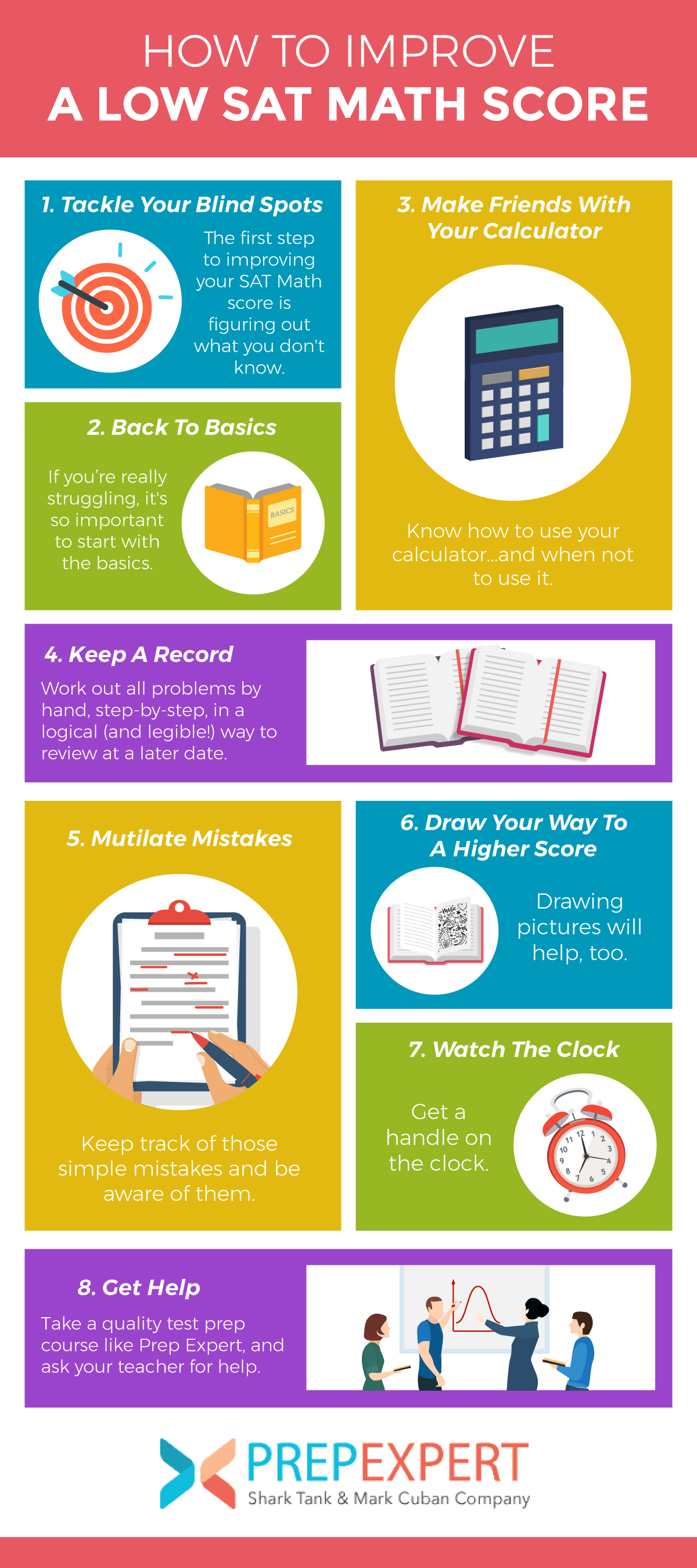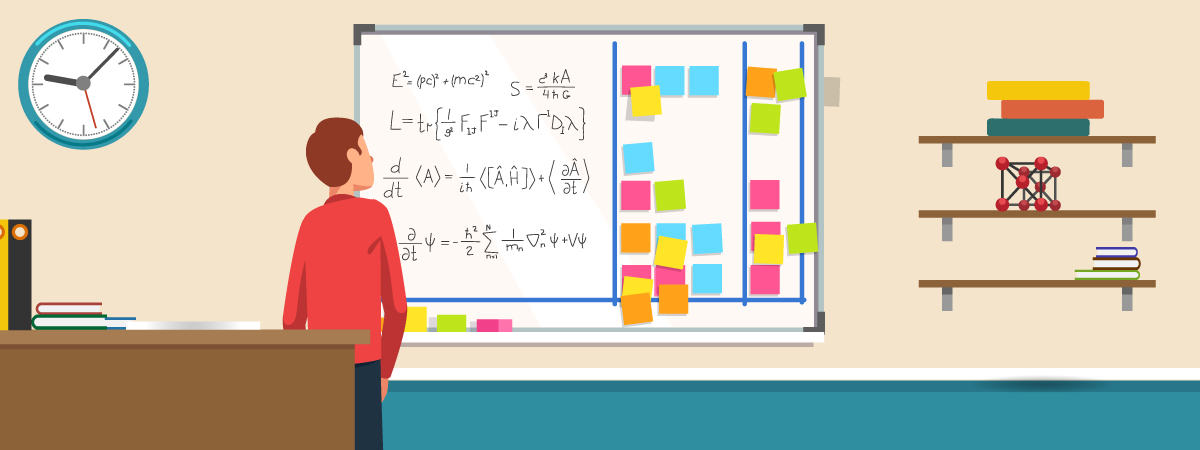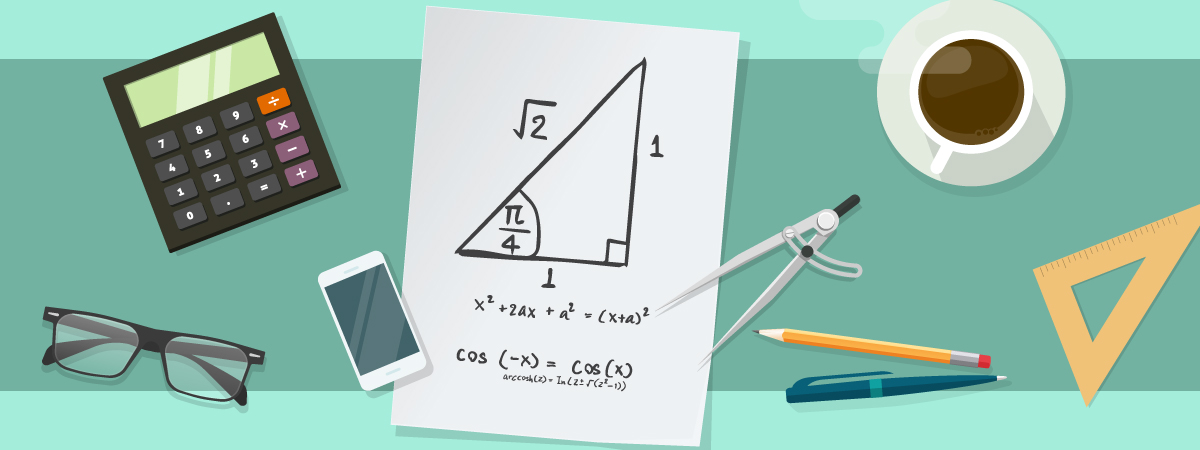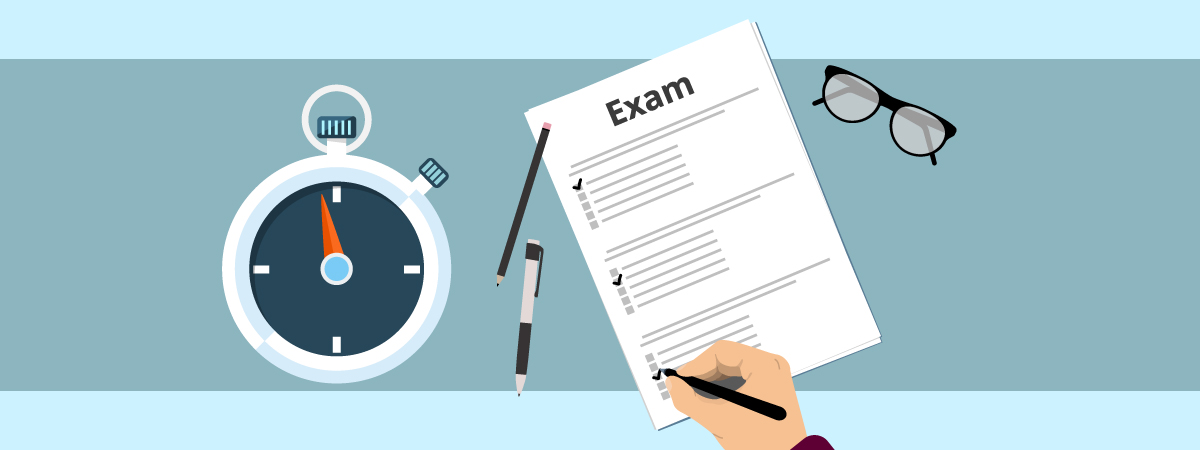# How To Improve A Low SAT Math Score

Whether you haven’t encountered some of the more advanced topics in school yet, or math is simply a difficult subject for you, there are plenty of things you can do to improve your SAT Math score. But like most things in life, it will take a lot of hard work to do so.

If you’re willing to keep track of your time with a journal, improve your understanding of calculator use, and start with the basics, you will almost surely improve your math score on SAT.

For specific help with the Math section of the SAT, check out our SAT prep course. Here’s how to get started.

[sat_one]The first step to improve your SAT Math score is figuring out what you don’t know. In order to do this you need to take a few practice tests — at least two each of the non-calculator and with-a-calculator sections — and figure out what types of questions you’re getting wrong.

To help you accomplish this, here’s a list of the types of topics on the SAT Math sections, along with the skills required for each of them, direct from the College Board.

You can use this list to mark off each type of problem as you encounter and then master it. A comprehensive study plan will be designed to cover each topic and type of problem until you’ve become familiar with, and confident in your ability to solve, all of them.

If you have any questions while reading, or want to speak with one of our test prep experts about our SAT prep courses, give us a call at (877) 345-7737 anytime!

## Heart Of Algebra

Topics

• Solving linear equations and linear inequalities (in these expressions, x is a constant or the product of a constant).
• Interpreting linear functions.
• Linear inequality and equation word problems.
• Graphing linear equations.
• Linear function word problems.
• Systems of linear inequalities word problems.
• Solving systems of linear equations.

Skills

• Use multiple steps to simplify an expression or equation or solve for a variable. Solve for a variable within functions or systems of inequalities with two variables (usually x and y).
• Determine whether a given point is in a solution set or what value would make an expression have no solution.
• Select a graph that shows an algebraic equation, or, on the flip side, choose the equation that describes a graph.
• Indicate how a graph would be affected by a given change in its equation.

Topics

• Interpreting nonlinear expressions.
• Quadratic and exponential word problems.
• Operations with rational expressions and polynomials.
• Polynomial factors and graphs.
• Nonlinear equation graphs.
• Structure in expressions.
• Isolating quantities.
• Functions.

Skills

• Solve equations by factoring or using other methods to rewrite them in another form.
• Add, subtract, multiply, or divide two rational expressions or divide two polynomial expressions and simplify your results.
• Select a graph that matches a nonlinear equation or an equation that corresponds to a graph.
• Determine the equation of a curve from a description of a graph.
• Figure out how a graph would change if its equation changed.

## Problem Solving & Data Analysis

Topics

• Ratios, rates, and proportions.
• Percentages.
• Units.
• Table data.
• Scatterplots.
• Key features of graphs.
• Linear and exponential growth.
• Data inferences.
• Center, spread, and shape of distributions.
• Data collection and conclusions.

Skills

• Solve multi-step problems to calculate ratio, rate, percentage, unit rate, or density.
• Use a given ratio, rate, percentage, unit rate, or density to solve a multistep problem.
• Select an equation that best fits a scatterplot.
• Use tables to summarize data, such as probabilities.
• Estimate populations based on sample data.
• Use statistics to determine mean, median, mode, range, and/or standard deviation.
• Evaluate tables, graphs, or text summaries.
• Determine the accuracy of a data collection method.

• Volume word problems.
• Right triangle word problems.
• Congruence and similarity.
• Right triangle geometry.
• Angles, arc lengths, and trig functions.
• Circle theorems.
• Circle equations.
• Complex numbers.

Skills

• Determine the volume of a shape.
• Apply properties of triangles to determine side length or angle measure.
• Apply properties of circles to measure arc length and area.
• Solve problems with sine, cosine, and tangent.

[sat_two]## Back To Basics

If you’re really struggling, it’s so important to start with the basics.

If you’re not doing well with the beginning, easier problems, or find that you’re making too many simple mistakes, then you might need to focus on the basics. Ask yourself, “what have I forgotten from freshman year, or even pre-high school, math?”

Re-acquaint yourself with simple terminology (such as, what is the difference between a real number and an integer?); concepts like the order of operations (PEMDAS, or, parenthesis, exponent, multiplication, division, addition, subtraction); and finding a common denominator.

Because most advanced math requires skill in basic math, each of these topics will serve as a building block for the others. So you’ll need these basic concepts down pat to build up to the more intense parts of the test.## Make Friends With Your Calculator

Know how to use your calculator…and when not to use it.

For Section 4 of the test, Math with Calculator, you’ll be able to use your graphing calculator to help solve problems. This is great—a good calculator will help you tackle problems with speed and efficiency. But in order to use the calculator well, you have to know how to use it.

There are lots of buttons and a multitude of functions on graphing calculators, so make sure that you sit down with your manual or math teacher and learn how to use them all. Learning how to make the most use out of these advanced machines is a lesson in and of itself.

In addition, be aware of the types of calculators that are and aren’t allowed on the exam. You aren’t allowed to use your iPhone calculator, nor can you use calculators with a computer algebra system, or CAS. When you purchase a calculator for the test, make sure you don’t buy one of these prohibited ones because you won’t be able to take it inside the test center!

Now, just because you have a calculator, doesn’t mean you should be dependent on it. You should learn how to solve all the problems by hand, with nothing more than a pencil and scratch paper. Learning this way not only ensures that you have a backup if your calculator malfunctions on test day, but also gives you the underlying confidence and skill you need to solve problems with ease and efficiency. Plus, you need to keep a record of your work!## Keep A Record

Work out all problems by hand, step-by-step, in a logical (and legible!) way to review at a later date.

In order to review problems you’ve completed to check for simple mistakes, you need to have a record of your work. If you do your work this way, you can spot whether you’ve forgotten to double a number when the question requires it, given an answer as a decimal rather than as a fraction, or given the measure of an angle in radians rather than degrees.## Mutilate Mistakes

Keep track of those simple mistakes and be aware of them.

As you take more and more practice tests, you’ll start to notice your “pet” mistakes—the types of mistakes that, for whatever reason, you make all the time. For some students, it’s forgetting to round up; or giving the answer for x when the question asks for a variation, like 2x or half of x; or maybe it’s mixing up the opposite and adjacent angles in a triangle.

If you learn what your pet mistakes are, you can easily look out for them on the exam. Then you’ll be able to remind yourself to check for them after completing a problem, and fix whatever needs correction.## Draw Your Way To A Higher Score

Drawing pictures will help, too.

Along with solving problems out, step-by-step, on paper, another surefire way to improve a low SAT math score is to get in the habit of drawing pictures whenever you can. Sometimes the SAT will provide figures along with questions, but when it doesn’t, there’s no stopping you from drawing one of your own. In almost all instances, this will make it easier to solve problems correctly.

For example, lots of questions related to volume or area can be solved by drawing a right triangle and using it to find a measure—such as height—that is missing from the information the question provides.

Drawing pictures will also help you make sense of problems, and figure out what steps you need to take to solve them when you’re stuck.## Watch The Clock

Get a handle on the clock.

One of the toughest parts of the SAT Math tests is finishing up in time. Remember that the questions on the math tests get harder as you go along, so you’ve got to spend less time on the beginning, easier questions than you do on the later, more difficult questions. The more practice tests you take, the better you will get at managing this time crunch.

A handy tip to keep in mind is that, if you’ve spent more than 10 seconds looking at a question without making any headway on it, then circle it and move on to the next question. Don’t make any single question the equivalent of your Titanic, and go down with the ship on a question that you’re not going to get right, losing the opportunity to answer plenty more that you would have gotten right.## Get Help

Take a quality test prep course like Prep Expert, and ask your teacher for help.

Maybe your problem is that you haven’t learned some of the more advanced SAT Math topics in school yet. Many students taking the SAT haven’t encountered trigonometry in class yet. If this is the case, and you’re getting those kinds of questions wrong, then the best thing to do is sign up for a test prep course, like Prep Expert.

You’ll then have access to qualified instructors who can teach you the math that you’re lacking. At school, you might be able to find a sympathetic math teacher who will take some time to teach you the topics you don’t know outside of class.

[sat_three]

For more test strategy, college admissions, and scholarship application tips sign up for our FREE class happening right now!